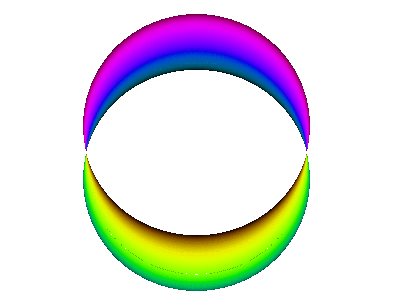# Question #d9e9d

Dec 27, 2016

The electron does not move in $\text{p}$ and $\text{d}$ orbitals.

#### Explanation:

The electron is not a little white ball with a minus sign whizzing around the orbitals in an atom.

Rather, it is behaving as a complicated 3-dimensional wave that has a "positive" amplitude in some places and simultaneously a "negative" amplitude in other places.

For example, here is a computer simulation of a standing wave. Notice how the colours in the two portions of the wave are always opposite colours.The electron waves in orbitals are something like that.

The pictures we see of orbitals show the regions where the wave has its greatest positive and negative amplitudes and, thus, where we find the greatest electron densities.

For example, in the $\text{3p}$ orbital below, the wave has opposite amplitudes in the brown and blue portions.In the same way, the electron waves in the $\text{3d}$ orbitals below have opposite amplitudes in the gold and red portions.(From fineartamerica.com)